# Poscat seminar 8

11 de May de 2016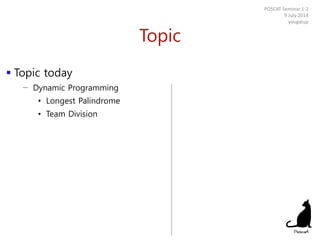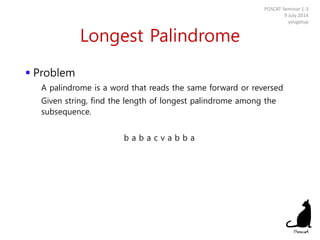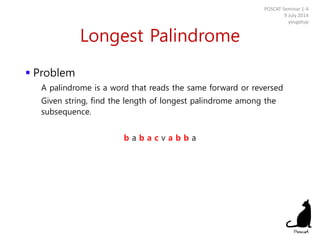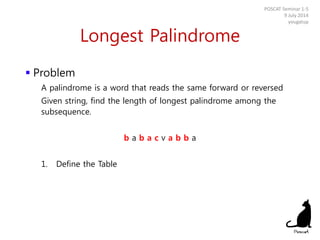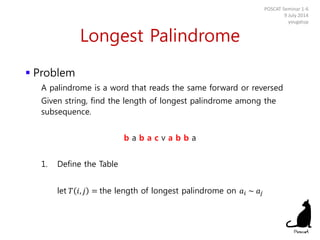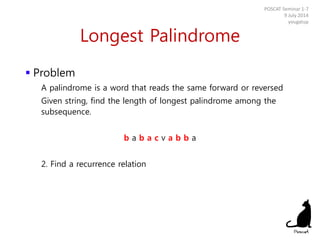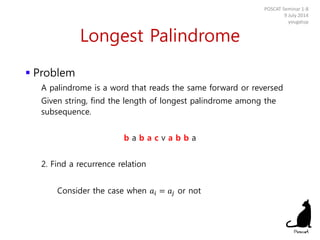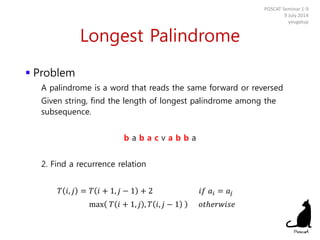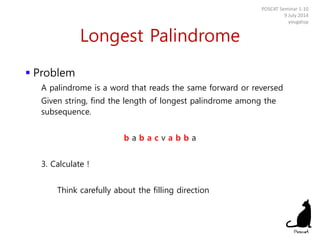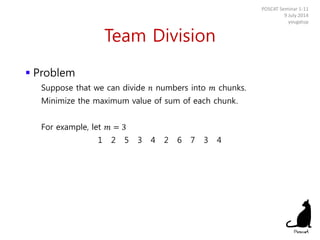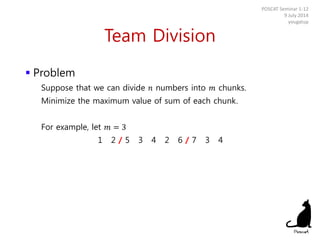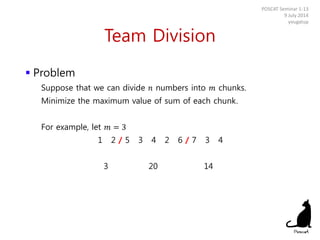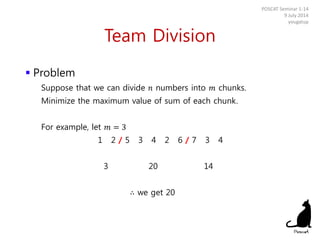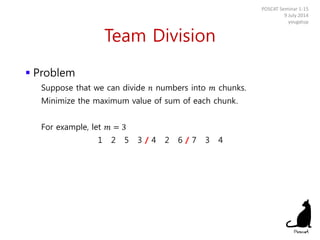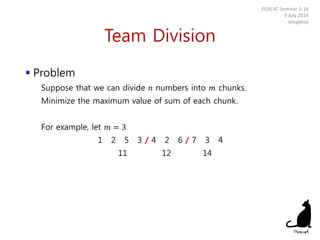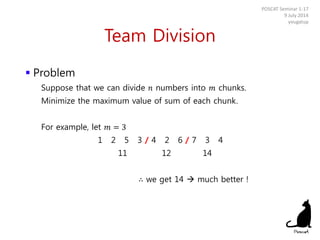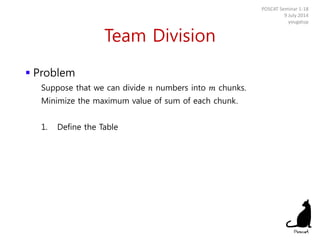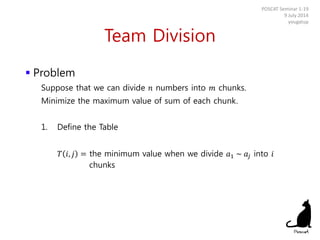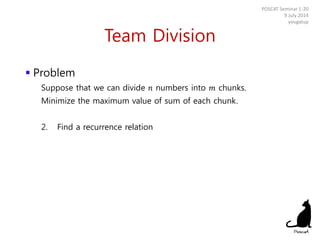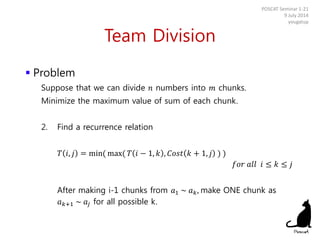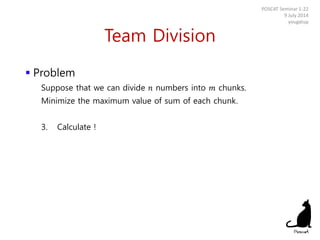1 de 22

### Poscat seminar 8

1. POSCAT Seminar 8 : Dynamic Programming 2 yougatup @ POSCAT 1
2. Topic  Topic today − Dynamic Programming • Longest Palindrome • Team Division POSCAT Seminar 1-2 9 July 2014 yougatup
3. Longest Palindrome  Problem A palindrome is a word that reads the same forward or reversed Given string, find the length of longest palindrome among the subsequence. b a b a c v a b b a POSCAT Seminar 1-3 9 July 2014 yougatup
4. Longest Palindrome  Problem A palindrome is a word that reads the same forward or reversed Given string, find the length of longest palindrome among the subsequence. b a b a c v a b b a POSCAT Seminar 1-4 9 July 2014 yougatup
5. Longest Palindrome  Problem A palindrome is a word that reads the same forward or reversed Given string, find the length of longest palindrome among the subsequence. b a b a c v a b b a 1. Define the Table POSCAT Seminar 1-5 9 July 2014 yougatup
6. Longest Palindrome  Problem A palindrome is a word that reads the same forward or reversed Given string, find the length of longest palindrome among the subsequence. b a b a c v a b b a 1. Define the Table let 𝑇 𝑖, 𝑗 = the length of longest palindrome on 𝑎𝑖 ~ 𝑎𝑗 POSCAT Seminar 1-6 9 July 2014 yougatup
7. Longest Palindrome  Problem A palindrome is a word that reads the same forward or reversed Given string, find the length of longest palindrome among the subsequence. b a b a c v a b b a 2. Find a recurrence relation POSCAT Seminar 1-7 9 July 2014 yougatup
8. Longest Palindrome  Problem A palindrome is a word that reads the same forward or reversed Given string, find the length of longest palindrome among the subsequence. b a b a c v a b b a 2. Find a recurrence relation Consider the case when 𝑎𝑖 = 𝑎𝑗 or not POSCAT Seminar 1-8 9 July 2014 yougatup
9. Longest Palindrome  Problem A palindrome is a word that reads the same forward or reversed Given string, find the length of longest palindrome among the subsequence. b a b a c v a b b a 2. Find a recurrence relation 𝑇 𝑖, 𝑗 = 𝑇 𝑖 + 1, 𝑗 − 1 + 2 𝑖𝑓 𝑎𝑖 = 𝑎𝑗 max 𝑇 𝑖 + 1, 𝑗 , 𝑇 𝑖, 𝑗 − 1 𝑜𝑡ℎ𝑒𝑟𝑤𝑖𝑠𝑒 POSCAT Seminar 1-9 9 July 2014 yougatup
10. Longest Palindrome  Problem A palindrome is a word that reads the same forward or reversed Given string, find the length of longest palindrome among the subsequence. b a b a c v a b b a 3. Calculate ! Think carefully about the filling direction POSCAT Seminar 1-10 9 July 2014 yougatup
11. Team Division  Problem Suppose that we can divide 𝑛 numbers into 𝑚 chunks. Minimize the maximum value of sum of each chunk. For example, let 𝑚 = 3 1 2 5 3 4 2 6 7 3 4 POSCAT Seminar 1-11 9 July 2014 yougatup
12. Team Division  Problem Suppose that we can divide 𝑛 numbers into 𝑚 chunks. Minimize the maximum value of sum of each chunk. For example, let 𝑚 = 3 1 2 / 5 3 4 2 6 / 7 3 4 POSCAT Seminar 1-12 9 July 2014 yougatup
13. Team Division  Problem Suppose that we can divide 𝑛 numbers into 𝑚 chunks. Minimize the maximum value of sum of each chunk. For example, let 𝑚 = 3 1 2 / 5 3 4 2 6 / 7 3 4 3 20 14 POSCAT Seminar 1-13 9 July 2014 yougatup
14. Team Division  Problem Suppose that we can divide 𝑛 numbers into 𝑚 chunks. Minimize the maximum value of sum of each chunk. For example, let 𝑚 = 3 1 2 / 5 3 4 2 6 / 7 3 4 3 20 14 ∴ we get 20 POSCAT Seminar 1-14 9 July 2014 yougatup
15. Team Division  Problem Suppose that we can divide 𝑛 numbers into 𝑚 chunks. Minimize the maximum value of sum of each chunk. For example, let 𝑚 = 3 1 2 5 3 / 4 2 6 / 7 3 4 POSCAT Seminar 1-15 9 July 2014 yougatup
16. Team Division  Problem Suppose that we can divide 𝑛 numbers into 𝑚 chunks. Minimize the maximum value of sum of each chunk. For example, let 𝑚 = 3 1 2 5 3 / 4 2 6 / 7 3 4 11 12 14 POSCAT Seminar 1-16 9 July 2014 yougatup
17. Team Division  Problem Suppose that we can divide 𝑛 numbers into 𝑚 chunks. Minimize the maximum value of sum of each chunk. For example, let 𝑚 = 3 1 2 5 3 / 4 2 6 / 7 3 4 11 12 14 ∴ we get 14  much better ! POSCAT Seminar 1-17 9 July 2014 yougatup
18. Team Division  Problem Suppose that we can divide 𝑛 numbers into 𝑚 chunks. Minimize the maximum value of sum of each chunk. 1. Define the Table POSCAT Seminar 1-18 9 July 2014 yougatup
19. Team Division  Problem Suppose that we can divide 𝑛 numbers into 𝑚 chunks. Minimize the maximum value of sum of each chunk. 1. Define the Table 𝑇 𝑖, 𝑗 = the minimum value when we divide 𝑎1 ~ 𝑎𝑗 into 𝑖 chunks POSCAT Seminar 1-19 9 July 2014 yougatup
20. Team Division  Problem Suppose that we can divide 𝑛 numbers into 𝑚 chunks. Minimize the maximum value of sum of each chunk. 2. Find a recurrence relation POSCAT Seminar 1-20 9 July 2014 yougatup
21. Team Division  Problem Suppose that we can divide 𝑛 numbers into 𝑚 chunks. Minimize the maximum value of sum of each chunk. 2. Find a recurrence relation 𝑇 𝑖, 𝑗 = min( max( 𝑇 𝑖 − 1, 𝑘 , 𝐶𝑜𝑠𝑡 𝑘 + 1, 𝑗 ) ) 𝑓𝑜𝑟 𝑎𝑙𝑙 𝑖 ≤ 𝑘 ≤ 𝑗 After making i-1 chunks from 𝑎1 ~ 𝑎 𝑘, make ONE chunk as 𝑎 𝑘+1 ~ 𝑎𝑗 for all possible k. POSCAT Seminar 1-21 9 July 2014 yougatup
22. Team Division  Problem Suppose that we can divide 𝑛 numbers into 𝑚 chunks. Minimize the maximum value of sum of each chunk. 3. Calculate ! POSCAT Seminar 1-22 9 July 2014 yougatup